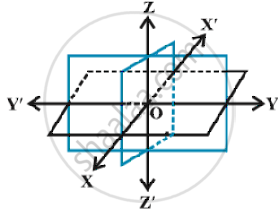# Three - Dimensional Geometry - Coordinate Axes and Coordinate planes

#### description

Coordinate Axes and Coordinate Planes in Three Dimensions

#### notes

Consider three planes intersecting at a point O such that these three planes are mutually perpendicular to each other .Fig.These three planes intersect along the lines X′OX, Y′OY and Z′OZ, called the x, y and z-axes, respectively.  These lines constitute the rectangular coordinate system. The planes XOY, YOZ and ZOX, called, respectively the XY-plane, YZ-plane and the ZX-plane, are known as the three coordinate planes.The XOY plane as the plane of the paper and the line Z′OZ as perpendicular to the plane XOY.
If the plane of the paper is considered as horizontal, then the line Z′OZ will be vertical. The distances measured from XY-plane upwards in the direction of OZ are taken as positive and those measured downwards in the direction of OZ′ are taken as negative.
Similarly, the distance measured to the right of ZX-plane along OY are taken as positive, to the left of ZX-plane and along OY′ as negative, in front of the YZ-plane along OX as positive and to the back of it along OX′ as negative. The point O is called the origin of the coordinate system and planes divide the space into eight parts known as octants. These octants could be named as XOYZ, X′OYZ, X′OY′Z, XOY′Z, XOYZ′, X′OYZ′, X′OY′Z′ and XOY′Z′.

If you would like to contribute notes or other learning material, please submit them using the button below.

### Shaalaa.com

Coordinate Axes and Coordinate Planes [00:10:11]
S
0%